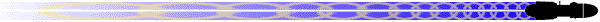# Tip O' The Day

 Calculations for resistors for use with LEDsCategory: Lighting Submitted By: Justin Miller Tip: Ok, you got your lighting set-up ready, and all the LEDs you need for the set-up...do you need resistors? You probably do...especially if you are using power from an outlet (but you still might need one even if using a 9V battery for example). There are two formulas you use to determine the following: 1) The Ohm rating of the resistor needed 2) The Wattage (rated for heat dissipation) of the resistor needed The first formula is Ohm's Law: V=I*R, where: V=Voltage I=Impedance (amps, or in the case of LEDs, milliamps) R=Resistance The formula is changed a bit since we are using LEDs and we are looking for R, and it's changed to this: R=(Vs-Vf)/If Again, R is Resistance, If is forward amps (stated, hopefully on the LED package or wherever you purchased them), Vs is source voltage (ex., 9V from a 9V battery) (sometimes stated as Vr for reverse voltage...same thing), and Vf is forward voltage (also from the package of the LED or wherever you purchased it from)...and use the Typical Vf and not the Maximum Vf. This sounds confusing but it really isn't. Here's an example: A green 3mm 3000mcd LED with a Vf of 3.6V and 20ma If that will be supplied power from a 5V source, therefore: R=(5-3.6)/0.02 R=1.4/0.02 R=70 Ohms Mind you there isn't a 70 Ohm resistor, so round UP to the next higher resistance value (in this case 75 Ohms), but if you didn't know it was 75 Ohms you needed, just remember the 70 and go to the next higher value when at Radio Shack or wherever. Now this gets a bit complicated with LEDs in parallel and series, but not to much. Only the following rules change, and apply to this formula and the next one: For parallel wiring: the If values are added together R=(Vs-Vf)/(If1+If2+...Ift) For serial wiring: the Vf values are added together R=(Vs-(Vf1+Vf2+...Vft)/If One more note...if Vf > Vs...you need a higher voltage supply, or nothing will light up since there isn't enough voltage for the circuit. Ok, you know the resistor value, but what wattage do you need? What would be overkill? That's what the next formula is for: Watt's Law. P=I*V P = Power in Watts, I and V carry over from the previous equation (and V will actually be (Vs-Vf) as before). This is simple multiplication at this point and this is rounded to the nearest 0.250. If P < 0.125, use a 1/8 watt resistor If P >= 0.125 and P < 0.25, use a 1/4 watt resistor If P >= 0.25 and P < 0.5, use a 1/2 watt resistor If P >= 0.5, use a 1 Watt resistor The tolerance level (the percentage number) is up to you, but I'd recommend 10% or better (meaning 10%, 5%, etc.). The proper resistor can mean the difference between a working LED circuit, blown LED(s) (or several) due to too much power being drawn by them, or LED(s) not lighting (either at all or not as bright as you want them to) due to not enough power reaching them.

 More Tips, Tricks and Techniques

Tips database last updated 21 July 2014

 Reader Tips: Search for tips on:    All Areas Budget Modeler Construction Painting Detailing Weathering Lighting Displaying Scratchbuilding Molds and Casting Other